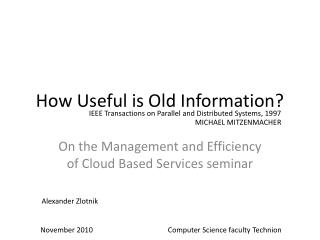DownloadDownload PresentationHow Useful is Old Information?

# How Useful is Old Information?

Download Presentation## How Useful is Old Information?

- - - - - - - - - - - - - - - - - - - - - - - - - - - E N D - - - - - - - - - - - - - - - - - - - - - - - - - - -
##### Presentation Transcript

1. How Useful is Old Information? IEEE Transactions on Parallel and Distributed Systems, 1997 MICHAEL MITZENMACHER On the Management and Efficiency of Cloud Based Services seminar Alexander Zlotnik November 2010 Computer Science faculty Technion

2. Amazon Elastic Load Balancing • Share Incoming Traffic • Scale Up • Scale Down • Detect &shutdown unhealthy EC2 instances • Balance single/multiple Availability Zones • Reports • \$0.025 per hour [\$18/month] + Traffic

3. Background • What is this paper not • Real system • Scale up/down system • Groups/AZ balancing

4. Background • Supermarket model & benefits • On incoming task, poll d queues for their load • Send the task to the shorter queue • For d = 2 the service time is exponentially shorter • Further d = 3, 4, … the improvement is linear

6. Setting • n nodes • Tasks Arrival: Poisson (λn) • Task is forwarded to a node for execution • Execution rate is distributed Exp(µ) • Normalized to µ=1 • Every T time units a bulletin board is updated with the current loads of all nodes

7. Policy • Look at drandom entries on the board, send the task to the shortest queue • d=1 • M/M/1 • d=n • Shortest queue  Static

8. Periodic updates 0 T 2T 3T Tt

9. Definitions • Pi,j(t) – Fraction of queues with posted load i, but have true load j • qi(t) - Rate of arrivals at a queue of size i Pi,j(t) True Load Posted Load

12. bi (t) – Fraction of queues with load i posted True Load Pi,j(t) Σ Posted Load

13. bi (t) – Fraction of queues with posted load i • Arrival rate to a queue with posted load i: • Tasks arrival • Chance that d selected queues have load i or more • Chance that a queue of size i is selected • Same chance for all queues of size i

14. Fixed Cycle • Hope: Convergence to a fixed point • State that there is no “motivation” to exit from • Periodic Updates • “Jump” on bulletin board updates, t=kT • Fixed cycle: bi do not change • Or, for k≥k0, P(kT) = P(k0T)

15. Fixed Cycle True Load Pi,j(t) Σ Posted Load Σ If π((k-1)T)= π(kT), the next phase will be the same

16. Fixed Cycle – finding vector π • Method A Run the system until changes in π are small • Method B mi,j(T) – Probability for M/M/1 queue to start with i tasks and after time T to have j tasks Solving a system of equations: • Method A on truncated system of differential equations

17. Fixed Cycle – finding vector π • Method B mi,j(T) – Probability for M/M/1 queue to start with i tasks and after time T to have j tasks • Solving a system of equations: Iterating π – Iteration end π – Iteration start x = • Until small changes in π

18. Fixed Cycle – finding vector π • Method B mi,j(T) – Probability for M/M/1 queue to start with i tasks and after time T to have j tasks • Iterating a system of equations: Bessel function of the first kind

19. Fixed Cycle – finding vector π • Method A on truncated system of differential equations • Bound I, J • Iterate on • Until small changes in π

20. Results

21. More complex Centralized Strategies • Time based • Split T into subintervals • At subinterval [tk,tk+1) tasks sent to random server with load at most k

22. More complex Centralized Strategies • Record-insert • Upon task arrival: • Sent to random server of servers with lowest load • Server’s load is incremented on bulleting board • Task ending not updated (until end of phase) • Uses real loads, not expectation • Always better than time-based

23. More complex Centralized Strategies

24. Continuous Update

25. Continuous Updates • Tasks benefit on occasional accurate info • Supported by other graphs too • Partial accurate info – not helpful • If not known which data is up to date